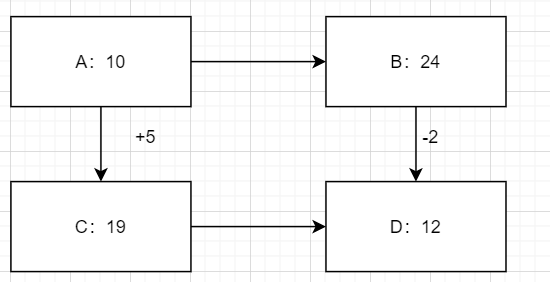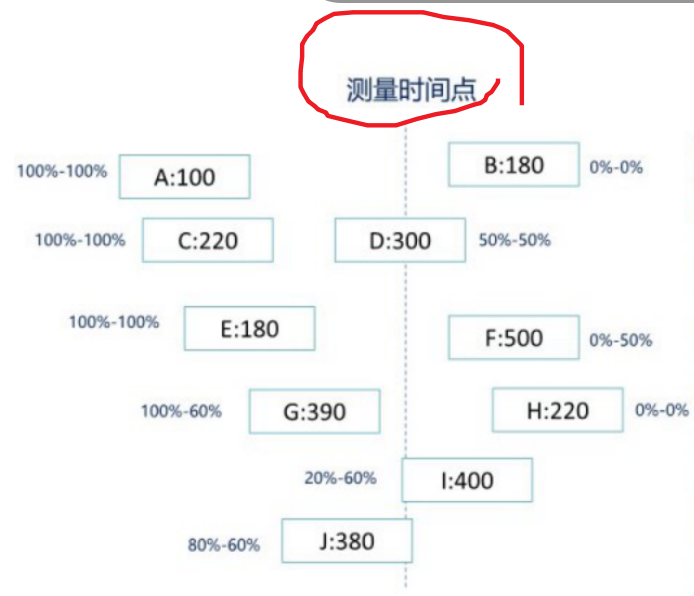# PMP 计算题整理

## 项目进度相关

### 关键路径法

A. 44 天
B. 48 天
C. 46 天### 估算活动时间

评价估算值 = （最乐观+最可能持续时间+最悲观）/ 3


活动历时均值(或估计值)=(乐观估计+4×最可能估计+悲观估计)/6
Te = (P + 4M + O)  / 6

-1δ~1δ                          68.26%
-2δ~2δ                          95.44%
-3δ~3δ                          99.72%
-6δ~6δ                          99.99%

(可能值+1个标准差)时间内完成的概率是(50%+(68.26%/2))=84.13%；
(可能值+2个标准差)时间内完成的概率是(50%+(95.44%/2))=97.72%；
(可能值+3个标准差)时间内完成的概率是(50%+(99.72%/2))=99.86%。


1）Te = (P + 4M + O)  / 6 = (7+4*4+3) / 6 = 4.33

2）SD = （P - O）/ 6 = 0.67

3）97.72% 在可能值+2个标准差上，4.33 + 0.67 * 2 = 5.67 天


（1）在16天内完成的概率是多少？
（2）在21天内完成的概率是多少？
（3）在21天之后完成的概率是多少？
（4）在21天到26天之间完成的概率是多少？
（5）在26天完成的概率是多少。

Te = (P + 4M + O)  / 6 = (36+4*21+6) / 6 = 21
SD = （P - O）/ 6 = (36-6)/6 = 5

1) 16 是 21 - 5 = 16，是均值减去一个方差的范围。因为 -1δ~1δ 的概率是 68.26%. 所以在该范围的概率是 1 -  68.26%=31.74%. 所以小于 16 天的概率是 31.74%/2=15.87%

2) 对于 21 天的均值来说，出现的概率是 50%. 正态分布的中心。

3) 1 - 50% = 50%

4) 在 -1δ~1δ 之内，是 68.26%

5）1 - (1 -  68.26%)/2 = 84.13%


## 项目成本相关

### 挣值管理PV （Planed Value）= 到某个时间点，所有活动加起来计划完成的工作量（价值）

EV （Earned Value）= 到某个时间点，所有活动加起来实际完成的工作量（价值）

AC （Actual Cost）= 到某个时间点，，所有活动加起来实际花费的钱数。

BAC （Budget at completion, 完工预算）= 完成整个项目，计算花费的资金

CV （Cost Variance）， 截止到某时点发生的实际成本与计划成本的偏差，CV = EV - AC

CV > 0 成本结余，反之成本超支。

CPI （Cost Performance Index，成本绩效指数）， 截止到某时点衡量成本绩效的一种指标，也就是实际每花一元钱，完成做了多少钱的事（花钱的效率），CPI=EV/AC

CPI > 0 效率高，超出计划的价值。反之，效率低。

SV（Schedule Variance）， 截止到某时点的实际进度与计划进度的偏差，**SV= EV - PV **

SV > 0 进度超前，反之进度落后。

SPI （Schedule Performance Index, 进度绩效指数 ），截止到某时点衡量进度绩效的一种指标，也就是实际完成的工作量与计划完成工作量之比，SPI=EV/PV

SPI > 0 超出工作量完成，反之小于工作量完成。

项目经理接一个工程项目，要建设 1.2 公里的铁路。根据以往的类似项目，预算

BAC = 100
PV = 100/1.2 * 6 = 50
EV = 100/1.2 * 6 = 50
AC = 60

CPI = EV/AC = 5/6 = 0.83 成本超支
SPI = EV/PV = 1 进度符合要求


ETC （Estimate to Completion，完工尚需估算）， 从某个时间点预测，剩余的工作还需要多少成本。

EAC（Estimate At Completion），在某个时间点，预测完成整个项目需要的成本。

ETC = BAC - EV

EAC = AC + ETC

1. 典型情况1 - 成本出现变化，进度不变：

ETC = (BAC - EV) / CPI

EAC = BAC / CPI = AC + ETC

例1： 项目预算工期为四天，分配的预算为 4000 美元。项目经理按每天 1000 美元分配一

1600 美元。

PV = 1000 * 2 = 2000
EV = 1600
CPI = EV/PV = 0.8
EAC = 4/0.8 = 5

EAC？()?

CPI = EV/AC=1000/1200
EAC = BAC/CPI=6000


2. 典型情况2 - 成本，进度都发生变化:

ETC = （BAC – EV）/（CPI x SPI）

EAC = AC + ETC

## 项目沟通相关

1、 计算沟通渠道：

公式：N * (N-1) / 2, N 为项目相关方数量，包含项目经理。



## 项目风险相关

EMV=20x20％+18x35％+(-20x15％)=3．3(万元)

EMV = 0.6 * 50000 + 0.35 * （-20000）=318000

## 参考

posted @ 2020-05-11 17:30  以终为始  阅读(...)  评论(...编辑  收藏